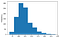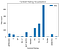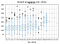# IMDB DataSet Visualization & Data Analytics Using Pandas

We have a .csv file of IMDB top 1000 movies and today we will be using this data to visualize and perform another type of analysis on it using Pandas.

I’m using Jupyter Notebook to add all of this code. So. first thing’s first, let’s import Pandas and Matplot libraries. Pandas to perform data analytics and Matplot for visualization.

`import pandas as pdimport matplotlib.pyplot as plt`

Read in ‘imdb_1000.csv’ and store it in a DataFrame named movies

`imdb_1000_data_url = r’data/imdb_1000.csv’movies = pd.read_csv(imdb_1000_data_url)movies.head() #to preview the data set`

Let’s get the initial idea about data

`# check the number of rows and columnsmovies.shape# check the data type of each columnmovies.dtypes`

Now that we know which column is of what data type we can perform operations on data like:

`# calculate the average movie durationmovies[‘duration’].mean()Out[]: 120.97957099080695`

The function we can perform on the data can be:

`# sort the DataFrame by duration to find the shortest and longest moviesmovies.sort_values(‘duration’)`

Let’s see the data in a visualization form.

`# create a histogram of duration, choosing an “appropriate” number of binsmovies[‘duration’].plot(kind=’hist’, bins=10)`This histogram tells us that most of the movies have a duration of 100–125 minutes

This is one of the benefits of using visualization for data that you can easily see the difference in data. There are different kinds of visualizations in which you can display the data. It’s up to your data type as there may be a case when the histogram is not telling you the right answer to the business question you are asking.

`# use a box plot to display that same datamovies[‘duration’].plot(kind=’box’)`

Box plot is telling us the same thing that most of the movies have a duration somewhere from 110 to 135 and we also have a clear median. Whereas, with histogram, we were unable to tell the median clearly.

Let’s do some more intermediate data analytics and visualizations using pandas.

`# count how many movies have each of the content ratingsmovies[[‘content_rating’,’title’]].groupby(‘content_rating’).count()`

To see this above data in a visualized form:

`# use a visualization to display that same data, including a title and x and y labelsmovies[[‘content_rating’,’title’]].groupby(‘content_rating’).count().plot(kind=’bar’, title=’Content Rating Visualization’)plt.xlabel(‘Content Rating’)plt.ylabel(‘Title Count’)`You choose title x and y label and many other properties to display data.

Here are some more analytics you can perform on the data.

`# convert the following content ratings to “NC-17”: X, TV-MAmovies[‘content_rating’].replace([‘X’,’TV-MA’],’NC-17')#.head()`

Let’s see if there is any missing value(s)

`# count the number of missing values in each columnmovies.isnull().sum(axis=0)# if there are missing values: examine them, then fill them in with “reasonable” valuesmovies[movies[‘content_rating’].isnull()]movies.at[(187,649),’content_rating’] = ‘PG’movies.at[936,’content_rating’] = ‘PG-13’`

You can also have inline conditions on data. For example;

`# calculate the average star rating for movies 2 hours or longer,# and compare that with the average star rating for movies shorter than 2 hoursprint(‘Avg. star rating for movies 2 hours or longer: ‘, movies[movies[‘duration’] >= 120][‘star_rating’].mean(),  ‘\nAvg. star rating for movies shorter than 2 hours: ‘, movies[movies[‘duration’] < 120][‘star_rating’].mean())`

Let’s do some visualization on this

`# use a visualization to detect whether there is a relationship between duration and star ratingmovies.boxplot(column=’duration’, by=’star_rating’);`This tells us that if the movie duration is below 125 mins it’s more likely to receive a rating.
`# calculate the average duration for each genremovies[[‘duration’,’genre’]].groupby(‘genre’).mean()`

Let’s visualize this data:

`# visualize the relationship between content rating and durationmovies.boxplot(column=’duration’, by=’content_rating’)`
`# determine the top rated movie (by star rating) for each genremovies.sort_values(‘star_rating’, ascending=False).groupby(‘genre’)[‘title’,’star_rating’].first()`

The first keyword is used to get the first value from a list/array or any sort of storage list type.

Some more analytics this data can give.

`# check if there are multiple movies with the same title, and if so, determine if they are actually duplicatesresult = movies[movies[‘title’].isin(movies[movies.duplicated([‘title’])][‘title’])]result.sort_values(‘title’)`

Inline condition:

`# calculate the average star rating for each genre, but only include genres with at least 10 moviesgenres = movies[‘genre’].value_counts()[movies[‘genre’].value_counts() > 10].indexmovies[movies[‘genre’].isin(genres)].groupby(‘genre’)[‘star_rating’].mean()`

Q: Figure out which actor did how many movies

We will start by cleaning the data:

`# Make a function which clean the datadef repp(string):    return string.replace("[","").replace("]","").replace("u'","").replace("',",",")[:-1]#Apply that function to every entry    movies_series = movies['actors_list'].apply(repp)#Declare a list to store the split valuesactors_list = []for movie_actors in movies_series:    actors_list.append([e.strip() for e in movie_actors.split(',')])#Declare a dictionary and see if the actor name key exist and then count accordingly.actor_dict = {}for actor in actors_list:    for a in actor:        if a in actor_dict:            actor_dict[a] +=1        else:            actor_dict[a] = 1actor_dict`

You can see all of this code on this Github Repository. Feel free to perform more functions on this data set as a practice.